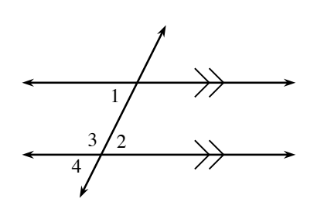### Home > GB8I > Chapter 6 Unit 7 > Lesson INT1: 6.3.3 > Problem6-110

6-110.

Refer to the diagram below.1. If $m∠1 = 74º$ and $m∠4 = 3x − 18º$, write an equation and solve for $x$.

$3x − 18 = 74$

$3x = 92$

$x = 30.6º$

1. If $m∠2 = 3x − 9º$ and $m∠1 = x + 25º$, write an equation and solve for $x$. Then determine $m∠2$.

Disregard your calculations for angle measure from part (a).

Since the lines are parallel, angles $1$ and $2$ have equal measure.

$x = 17º$, $m∠2=42º$